Author: Oscar Cronquist Article last updated on February 15, 2022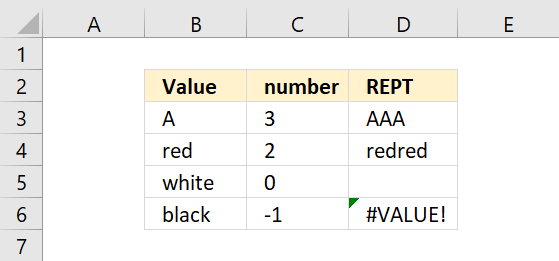The REPT function repeats a specific text a chosen number of times.

Formula in cell D3:

=REPT(B3,C3)

## 1. REPT Function Syntax

REPT(text, number_times)

## 2. REPT Function Arguments

 text Required. The value you want to repeat. number_times Required. The number of times you want to repeat the value.

## 3. REPT function error

• 0 (zero) makes the REPT function return nothing.
• A negative number or a text string returns a #VALUE error.

## 4. REPT function bar chart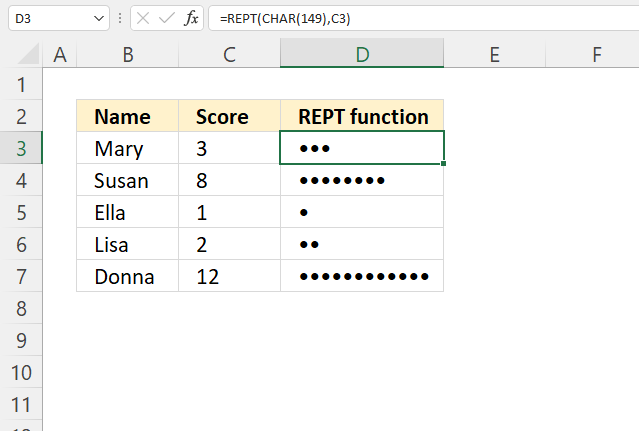The image above demonstrates how to create a basic bar chart using the REPT function. It repeats any character or symbol you like letting you quickly compare numbers.

Formula in cell D3:

=REPT(CHAR(149),C3)

### 4.1 Explaining formula

#### Step 1 - Create a large dot

The CHAR function returns a character or symbol based on a number between 1 and 255.

CHAR(text)

CHAR(149)

returns •

#### Step 2 - Repeat large dot based on given number

REPT(CHAR(149),C3)

becomes

REPT("•",C3)

becomes

REPT("•",3)

and returns "•••".

## 5. REPT function alternative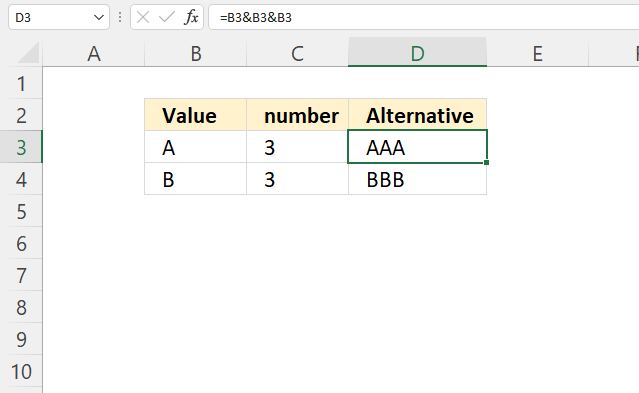The ampersand character concatenates values, this can be used to repeat values.

Formula in cell D3:

=B3&B3&B3

The formula in cell D3 concatenates the specified value in cell B3 three times, in other words, repeating the value three times.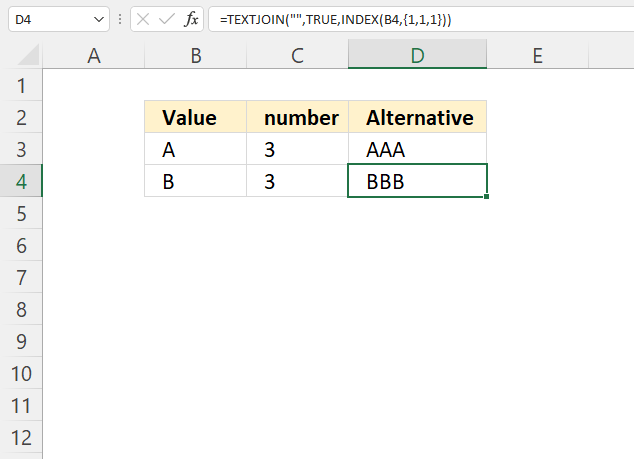Formula in cell D4:

=TEXTJOIN("",TRUE,INDEX(B4,{1,1,1}))

### 5.1 Explaining formula

#### Step 1 - Create array

The curly brackets let you create an array of values in an Excel formula. The comma and semicolon are delimiting characters you can use, the comma separates values horizontally or column-wise. The semicolon delimits values vertically or row-wise.

The comma and semicolon are determined by your computer's regional settings.

{1,1,1}

#### Step 2 - Repeat value in cell B4

The INDEX function gets a value from a cell range or array based on a row and column number (optional).

INDEX(B4,{1,1,1})

becomes

INDEX("B",{1,1,1})

and returns {"B", "B", "B"}.

#### Step 3 - Join values in the array

The TEXTJOIN function concatenates values.

TEXTJOIN(delimiterignore_emptytext1[text2], ...)

TEXTJOIN("",TRUE,INDEX(B4,{1,1,1}))

becomes

TEXTJOIN("",TRUE,{"B", "B", "B"})

and returns "BBB".

## 6. REPT function array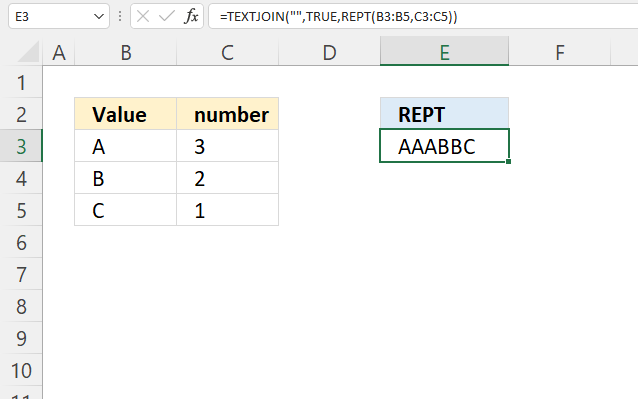Formula in cell E3:

=TEXTJOIN("", TRUE, REPT(B3:B5, C3:C5))

### 6.1 Explaining formula

#### Step 1 - Repeat values in an array based on corresponding numbers on the same row

REPT(B3:B5,C3:C5)

becomes

REPT({"A"; "B"; "C"}, {"3"; "2"; "1"})

and returns

{"AAA"; "BB"; "C"}

#### Step 2 - Join values without a delimiter

The TEXTJOIN function concatenates values.

TEXTJOIN(delimiterignore_emptytext1[text2], ...)

TEXTJOIN("",TRUE,REPT(B3:B5,C3:C5))

becomes

TEXTJOIN("",TRUE,{"AAA"; "BB"; "C"})

and returns

"AAABBC".

## 7. REPT function based on list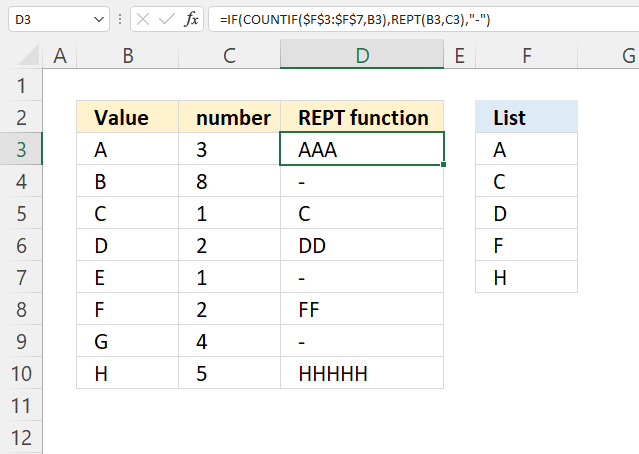The image above shows a formula in cell D3 that repeats the value in cell B3 if it exists in the list in F3:F7.

=IF(COUNTIF(\$F\$3:\$F\$7,B3),REPT(B3,C3),"-")

### 7.1 Explaining formula

#### Step 1 - Repeat value

REPT(B3,C3)

becomes

REPT("A", 3)

and returns "AAA".

#### Step 2 - Check if value is in the list

The COUNTIF function counts the number of cells that is equal to a condition.

COUNTIF(rangecriteria)

COUNTIF(\$F\$3:\$F\$7,B3)

becomes

COUNTIF({"A"; "C"; "D"; "F"; "H"},"A")

and returns 1. Cell value "A" is found once in the list.

#### Step 3 - Evaluate IF function

The IF function returns one value if the logical test is TRUE and another value if the logical test is FALSE.

IF(logical_test, [value_if_true], [value_if_false])

IF(COUNTIF(\$F\$3:\$F\$7,B3),REPT(B3,C3),"-")

becomes

IF(1, REPT(B3, C3), "-")

becomes

IF(1, REPT("A", 3), "-")

becomes

IF(1, "AAA", "-")

and returns "AAA".

## 8 REPT function based on list and concatenated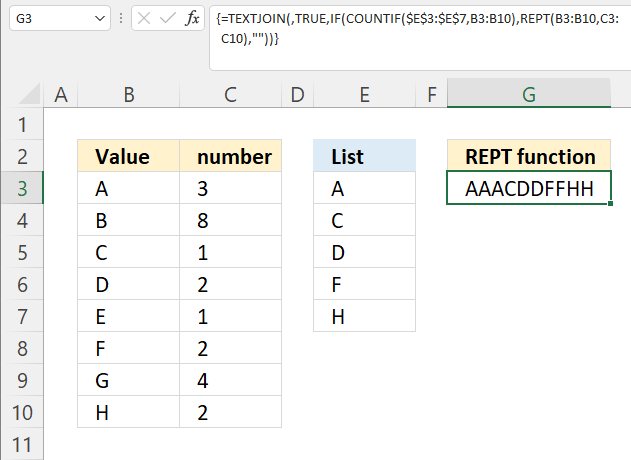Formula in cell G3:

=TEXTJOIN(,TRUE,IF(COUNTIF(\$E\$3:\$E\$7,B3:B10),REPT(B3:B10,C3:C10),""))

### 8.1 Explaining formula

#### Step 1 - Repeat value

REPT(B3:B10, C3:C10)

becomes

REPT({"A"; "B"; "C"; "D"; "E"; "F"; "G"; "H"},{3; 8; 1; 2; 1; 2; 4; 2})

and returns

{"AAA"; "BBBBBBBB"; "C"; "DD"; "E"; "FF"; "GGGG"; "HH"}.

#### Step 2 - Check if value is in the list

The COUNTIF function counts the number of cells that is equal to a condition.

COUNTIF(rangecriteria)

COUNTIF(\$F\$3:\$F\$7,B3:B10)

becomes

COUNTIF({"A"; "C"; "D"; "F"; "H"}, {"A"; "B"; "C"; "D"; "E"; "F"; "G"; "H"})

and returns

{1; 0; 1; 1; 0; 1; 0; 1}

#### Step 3 - Evaluate IF function

The IF function returns one value if the logical test is TRUE and another value if the logical test is FALSE.

IF(logical_test, [value_if_true], [value_if_false])

IF(COUNTIF(\$F\$3:\$F\$7,B3),REPT(B3,C3),"")

becomes

IF({1; 0; 1; 1; 0; 1; 0; 1}, {"AAA"; "BBBBBBBB"; "C"; "DD"; "E"; "FF"; "GGGG"; "HH"},"")

and returns

{"AAA"; ""; "C"; "DD"; ""; "FF"; ""; "HH"}

#### Step 4 - Concatenate values in array

The TEXTJOIN function allows you to combine text strings from multiple cell ranges and also use delimiting characters if you like.

TEXTJOIN(delimiterignore_emptytext1[text2], ...)

TEXTJOIN(,TRUE,IF(COUNTIF(\$E\$3:\$E\$7,B3:B10),REPT(B3:B10,C3:C10),""))

becomes

TEXTJOIN(,TRUE,{"AAA"; ""; "C"; "DD"; ""; "FF"; ""; "HH"})

and returns "AAACDDFFHH".